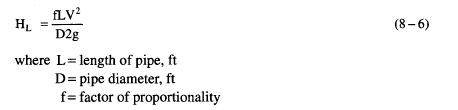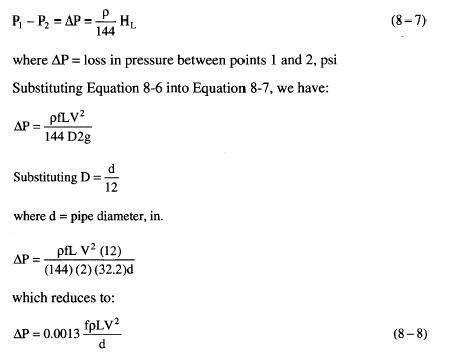# Piping – Darcy's Equation

This equation, which is also sometimes called the Weisbach equation or the Darcy-Weisbach equation, states that the friction head loss between two points in a completely filled, circular cross section pipe is proportional to the velocity head and the length of pipe and inversely proportional to the pipe diameter. This can be written:Equations 8-5 and 8-6 can be used to calculate the pressure at any point in a piping system if the pressure, flow velocity, pipe diameter, and elevation are known at any other point. Conversely, if the pressure, pipe diameter, and elevations are known at two points, the flow velocity can be calculated.

In most production facility piping systems the head differences due to elevation and velocity changes between two points can be neglected. In this case Equation 8-5 can be reduced to: